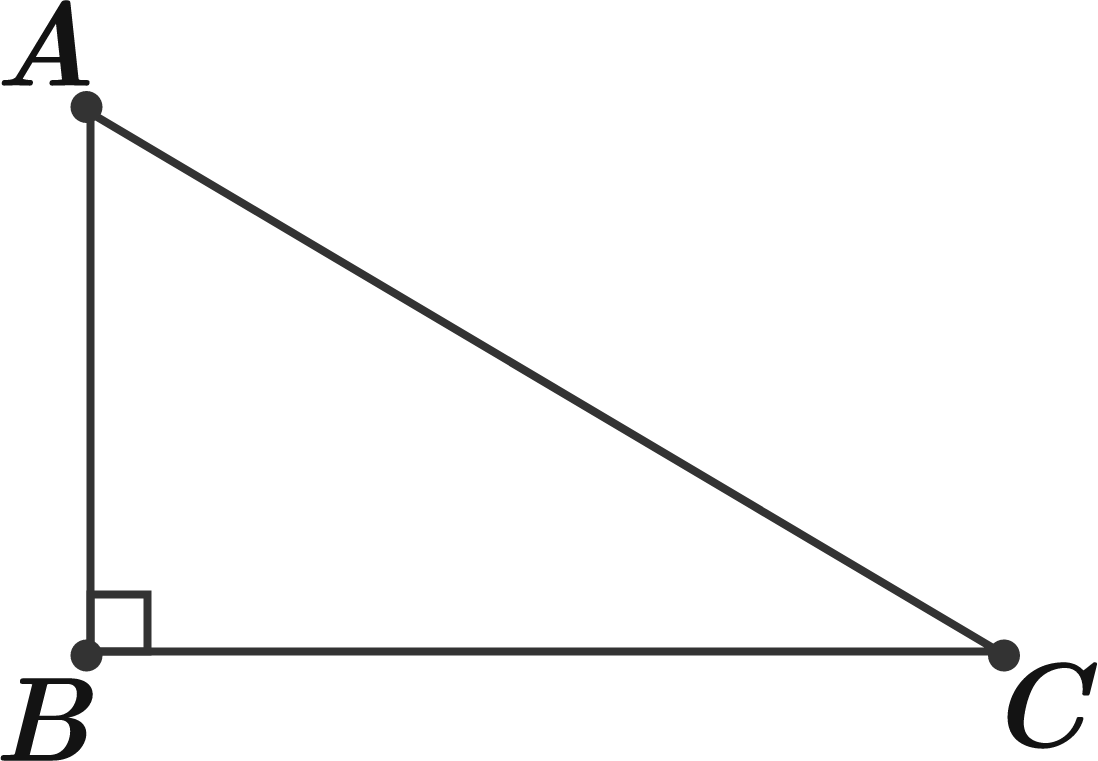# Geometric Geometry

Geometry Level 1

If the lengths of sides $AB,$ $BC$ and $AC$ in the figure shown form a geometric progression in that order, what is the ratio between $AC$ and $AB$ to 3 decimal places?×